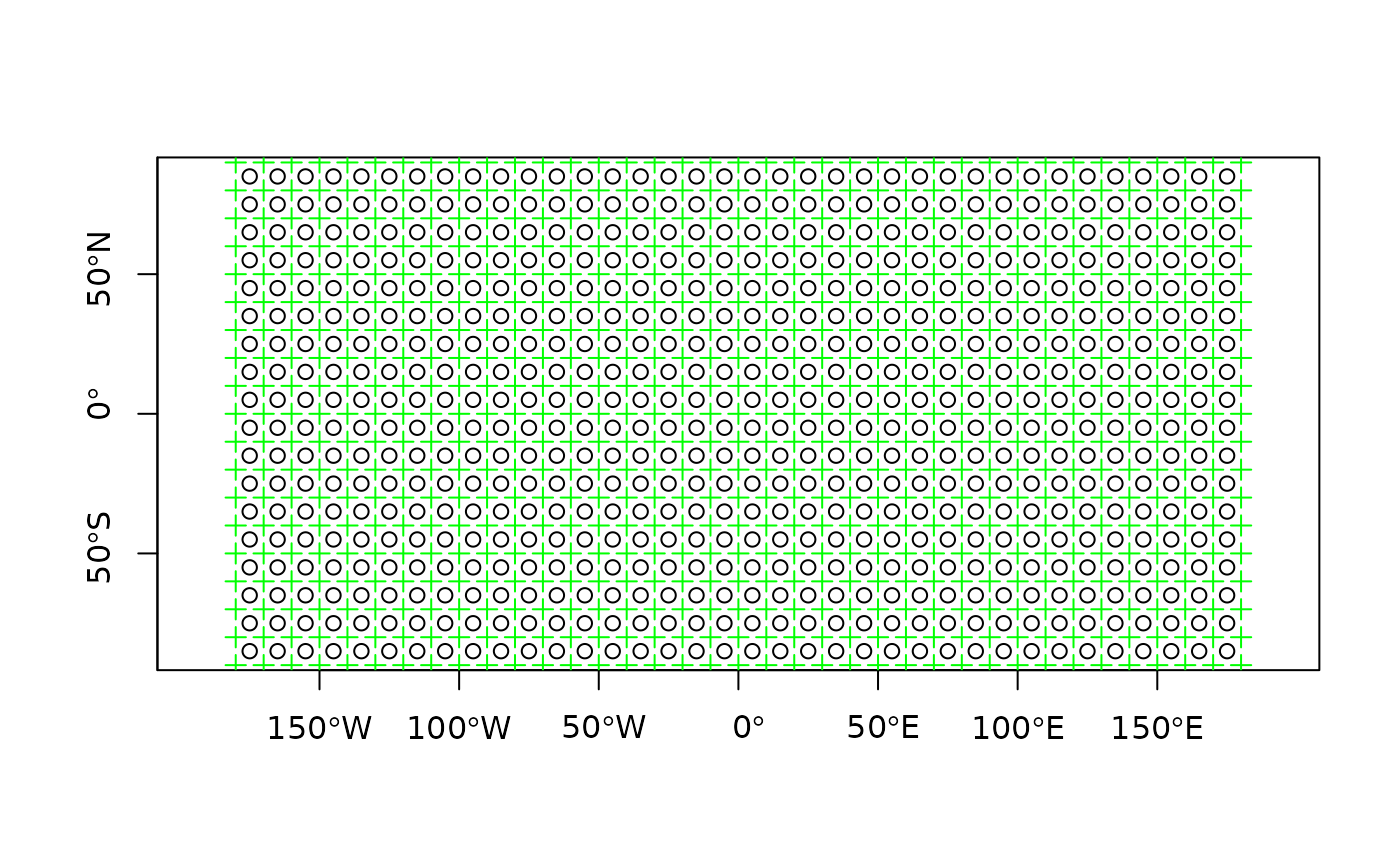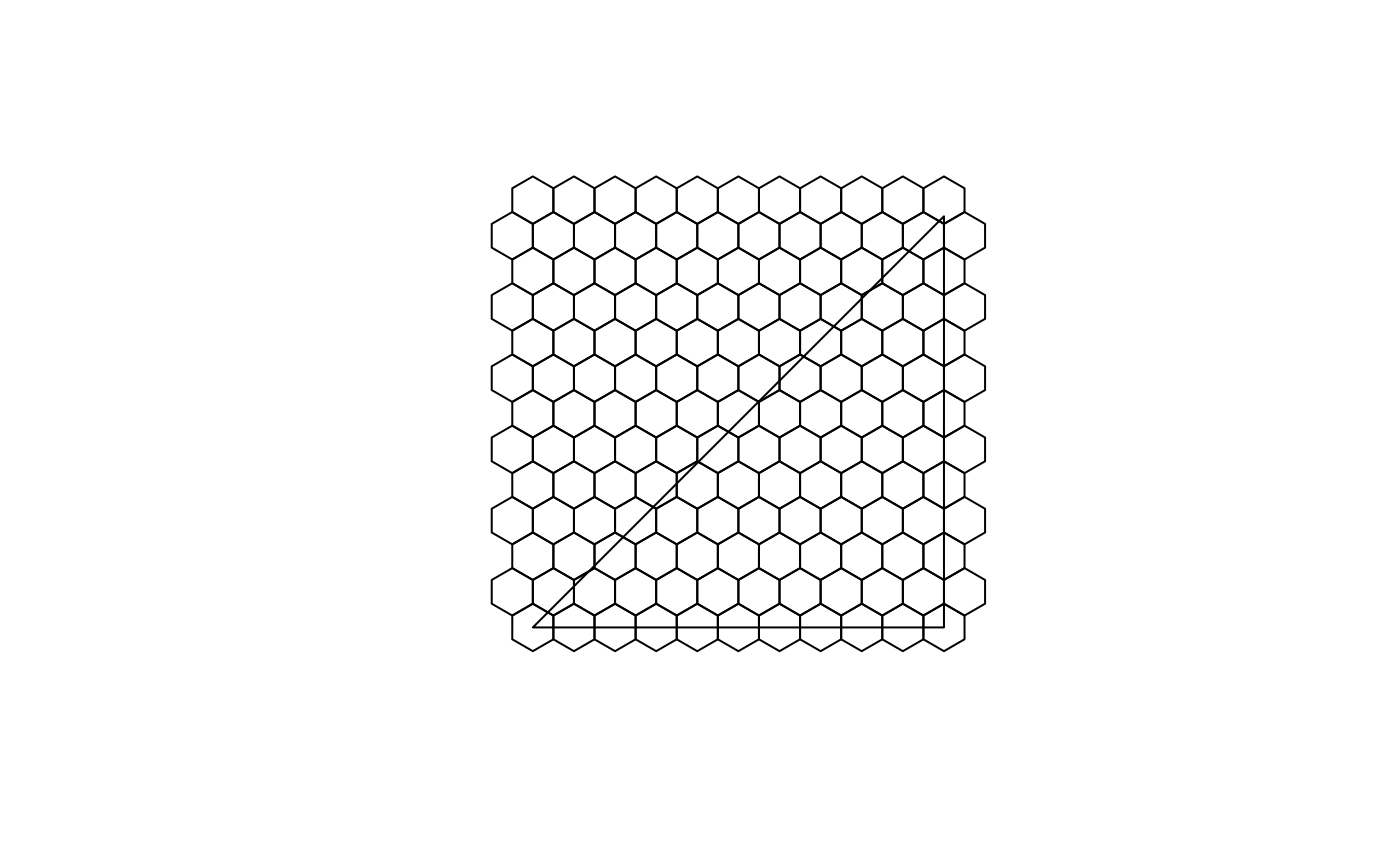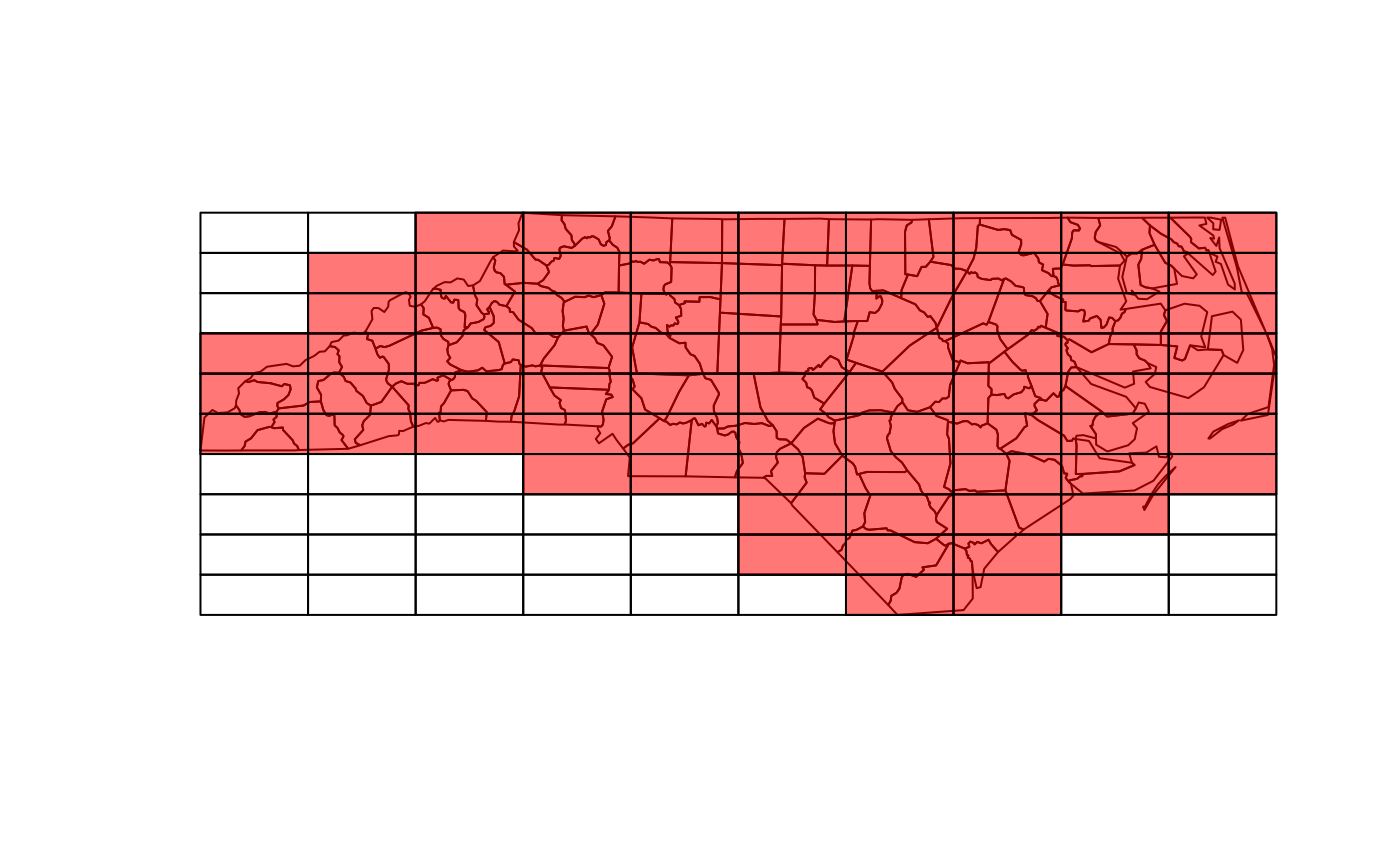Create a square or hexagonal grid covering the bounding box of the geometry of an sf or sfc object

## Usage

st_make_grid(
x,
cellsize = c(diff(st_bbox(x)[c(1, 3)]), diff(st_bbox(x)[c(2, 4)]))/n,
offset = st_bbox(x)[c("xmin", "ymin")],
n = c(10, 10),
crs = if (missing(x)) NA_crs_ else st_crs(x),
what = "polygons",
square = TRUE,
flat_topped = FALSE
)

## Arguments

x

object of class sf or sfc

cellsize

numeric of length 1 or 2 with target cellsize: for square or rectangular cells the width and height, for hexagonal cells the distance between opposite edges (edge length is cellsize/sqrt(3)). A length units object can be passed, or an area unit object with area size of the square or hexagonal cell.

offset

numeric of length 2; lower left corner coordinates (x, y) of the grid

n

integer of length 1 or 2, number of grid cells in x and y direction (columns, rows)

crs

object of class crs; coordinate reference system of the target of the target grid in case argument x is missing, if x is not missing, its crs is inherited.

what

character; one of: "polygons", "corners", or "centers"

square

logical; if FALSE, create hexagonal grid

flat_topped

logical; if TRUE generate flat topped hexagons, else generate pointy topped

## Value

Object of class sfc (simple feature geometry list column) with, depending on what and square, square or hexagonal polygons, corner points of these polygons, or center points of these polygons.

## Examples

plot(st_make_grid(what = "centers"), axes = TRUE)
plot(st_make_grid(what = "corners"), add = TRUE, col = 'green', pch=3)sfc = st_sfc(st_polygon(list(rbind(c(0,0), c(1,0), c(1,1), c(0,0)))))
plot(st_make_grid(sfc, cellsize = .1, square = FALSE))# non-default offset:
plot(st_make_grid(sfc, cellsize = .1, square = FALSE, offset = c(0, .05 / (sqrt(3)/2))))#> Reading layer nc' from data source
#>   /home/runner/work/_temp/Library/sf/shape/nc.shp' using driver ESRI Shapefile'
#> Simple feature collection with 100 features and 14 fields
#> Geometry type: MULTIPOLYGON
#> Dimension:     XY
#> Bounding box:  xmin: -84.32385 ymin: 33.88199 xmax: -75.45698 ymax: 36.58965`